# Facts and Figures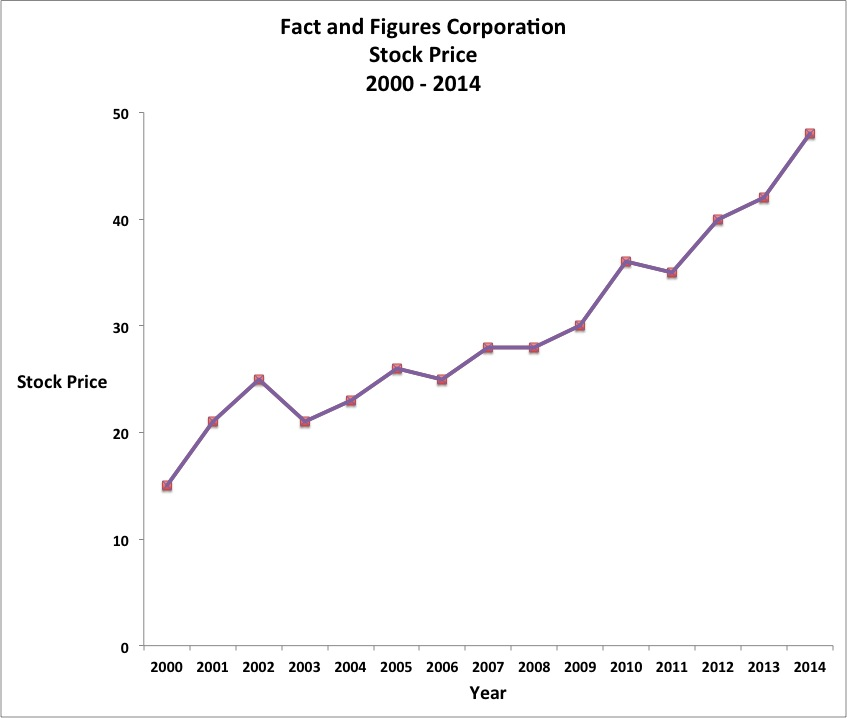Basics
Although data will be depicted in various ways in the Mathematical Reasoning module, you will run across different displays of data in other GED modules, too.

Among the ways numerical facts can be rendered in pictorial figures are:
Line Graph
• specific, connected data

Scatter Plot
• specific, unconnected data

Bar Chart
• specific, columnar data

Histogram
• range of columnar data

Pie Chart
• slices of data

Dot Plot
• specific, dotted data

The data in the table below is used to formulate the line graph, scatter plot, bar chart, histogram, pie chart, and dot plot that follow.

 Facts and Figures Corporation Year Stock Price 2000 15 2001 21 2002 25 2003 21 2004 23 2005 26 2006 25 2007 28 2008 28 2009 30 2010 36 2011 35 2012 40 2013 42 2014 48

Line Graph (specific, connected data)Scatter Plot (specific, unconnected data)Bar Chart (specific, columnar data)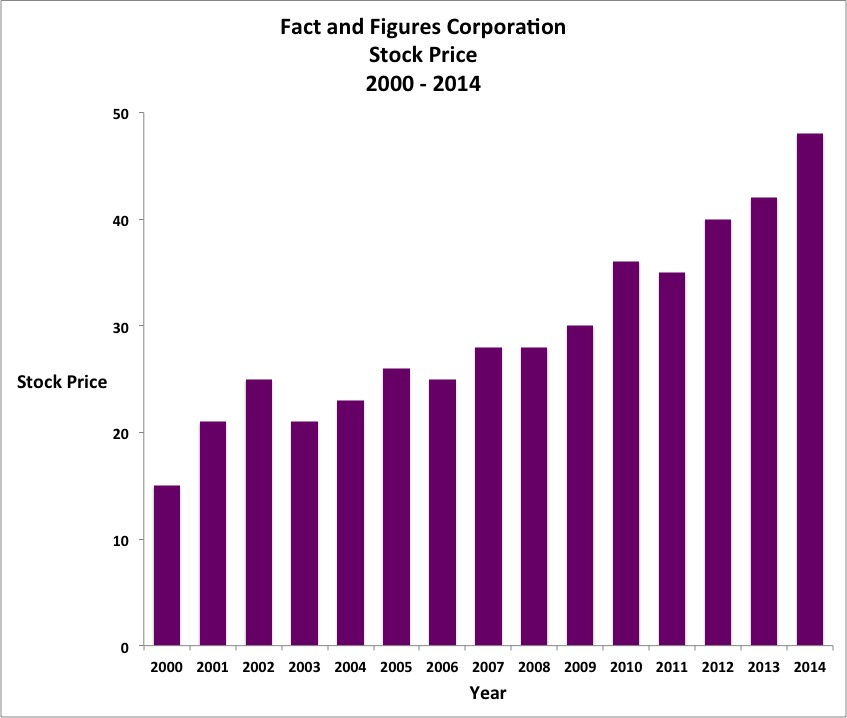Histogram (range of columnar data)Pie Chart (slices of data)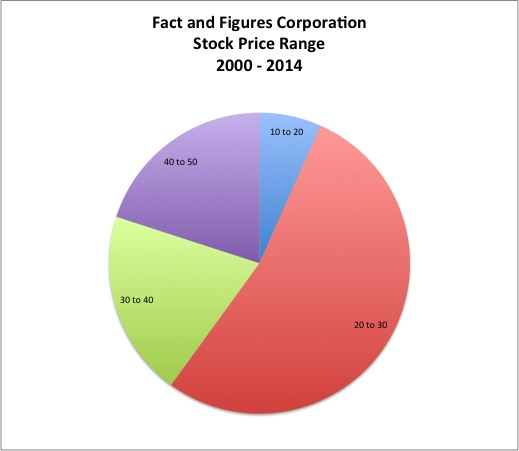Dot Plot (specific, dotted data)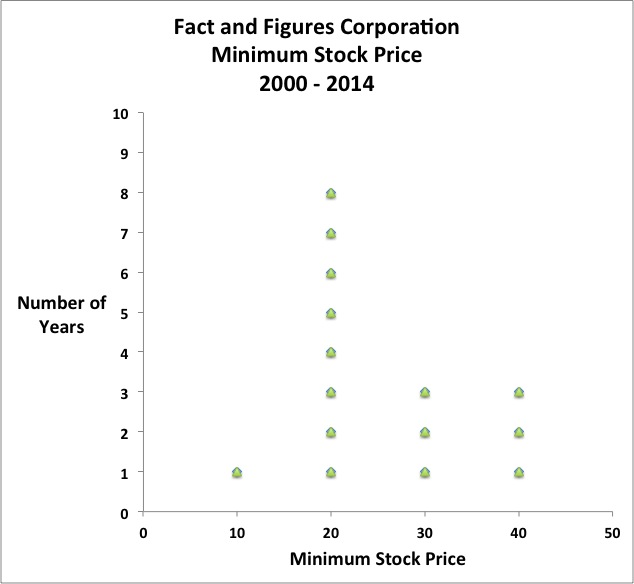Question – Science ModuleSome researchers claim that the price of a stock is correlated with the number of mentions a stock receives in the financial news media.

If that research is true, between what years in the line graph above did the number of mentions of  the stock of Facts and Figures Corporation decline the most in the financial news media?
A.  2005 – 2006
B.  2009 – 2010
C.  2007 – 2008
D.  2002 – 2003

D.  2002 – 2003

According to the line graph, the steepest decline in the price of the stock of Facts and Figures Corporation occurred between the years 2002 and 2003.  If the research is true, that time period should correlate with the fewest number of mentions of the stock in the financial news media.

Question – Social Studies ModuleIt has long been said that the price of a stock is a function of the human frailties of fear and greed.

If that theory is true, in what price range of the stock of Facts and Figures Corporation in the histogram above were investors most likely to be paralyzed between fear and greed?
A.  10 – 20
B.  20 – 30
C.  30 – 40
D.  40 – 50

B.  20 – 30

According to the histogram, the price of the stock of Facts and Figures Corporation was stuck in a range between 20 and 30 for 8 years.  That lengthy period of minimal price movement suggests that investors themselves were stuck between fear and greed.

Question – Reasoning Through Language Arts ModuleThe stock market has long been the butt of many famous jokes as follows:

“I never attempt to make money on the stock market.  I buy on the assumption that they could close the market the next day and not reopen it for five years.” — Warren Buffett

“One of the funny things about the stock market is that every time one person buys, another sells, and both think they are astute.” — William Feather

“I am more concerned about the return of my money than the return on my money.” — Mark Twain and/or Will Rogers

At what price in the dot plot above would you infer that the joke-tellers would feel most comfortable owning stock in Facts and Figures Corporation?
A.  20
B.  30
C.  40
D.  50

A.  20

According to the dot plot, the price of the stock of Facts and Figures Corporation is most likely to maintain a minimum price of 20.  That price would be most in keeping with the conservative stance of the joke-tellers.

Question – Mathematical Reasoning ModuleUsing the data from the the scatter plot above, which of the following represents the
slope-intercept form of the equation of a line passing through the points at the year 2000 and the year 2009?

A.  y = 1.67x + 3325
B.  y = -1.67x + 3325
C.  y = -1.67x – 3325
D.  y = 1.67x – 3325

D.  y = 1.67x – 3325

See Slope-Intercept.

(x1, y1) = (2000, 15)
(x2, y2) = (2009, 30)

slope of a line$\bf\displaystyle m=\frac{{{y}_{2}}-{{y}_{1}}}{{{x}_{2}}-{{x}_{1}}}$$\bf\displaystyle m =\frac{30-15}{2009- 2000}$$\bf\displaystyle\frac{5}{3}$ = 1.666666667 after toggle = 1.67 after rounding

slope-intercept b$\bf\displaystyle b=y-mx$
(x1, y1) or (x2, y2) can be used to calculate b; in this case, (x1, y1) = (2000, 15) will be used:$\bf\displaystyle b=y-mx$ = 15 – 1.67 × 2000 = -3325

slope-intercept form of the equation of a line$\bf\displaystyle y=mx+b$
y = 1.67x – 3325

Calculator Click What You See Commentblinker clears screen30 – 15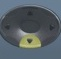2009 – 2000$\bf\displaystyle m =\frac{30-15}{2009- 2000}$$\bf\displaystyle m=\frac{{{y}_{2}}-{{y}_{1}}}{{{x}_{2}}-{{x}_{1}}}$$\bf\displaystyle \frac{5}{3}$1.666666667 m (before rounding)
15 – 1.67 × 2000 15-1.67*2000$\bf\displaystyle b=y-mx$-3325 b